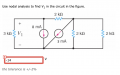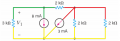# Nodal Analysis Question

#### Norbino

Joined Mar 8, 2020
7
Hello,
The concept of nodal analysis is clear to me, however in some circuits I get very confused when there is one element which I have not seen before/the circuit is not basic. There are two questions I have, one is how many nodes are there in this circuit/where they are, and two, for writing the expression for the current leaving/entering pertaining to the 2 kOhm resistor on the top when to use the form (V1-V2)/R or simply V2/R. I have attached the circuit in question and also attached a screenshot of how I obtained -14V.#### WBahn

Joined Mar 31, 2012
29,167
You seem to have identified the nodes just fine. A simple way to identify the nodes is to color code them. Pick a color and the overlay it on a particular wire everywhere right up to where it enters a component. Every component touching that color is connected to that node.So you have three nodes. You get to arbitrarily pick one of them and call it zero volts. You picked the yellow node (which is a very reasonable choice).

As for your second question, Ohm's Law is ALWAYS the voltage difference ACROSS the resistor (Va relative to Vb) and the current THROUGH that same resistor (from Node a to Node b).

So it is intrinsically

$$I_{ab} \; = \; \frac{V_{ab}}{R} \; = \; \frac{V_a \; - \; V_b}{R}$$

With that in mind, go back and redo your node equations.

•Norbino

#### Norbino

Joined Mar 8, 2020
7
You seem to have identified the nodes just fine. A simple way to identify the nodes is to color code them. Pick a color and the overlay it on a particular wire everywhere right up to where it enters a component. Every component touching that color is connected to that node.
View attachment 201026

So you have three nodes. You get to arbitrarily pick one of them and call it zero volts. You picked the yellow node (which is a very reasonable choice).

As for your second question, Ohm's Law is ALWAYS the voltage difference ACROSS the resistor (Va relative to Vb) and the current THROUGH that same resistor (from Node a to Node b).

So it is intrinsically

$$I_{ab} \; = \; \frac{V_{ab}}{R} \; = \; \frac{V_a \; - \; V_b}{R}$$

With that in mind, go back and redo your node equations.
Wonderful, I see where I went wrong with my previous solution and I got the correct answer now. The technique of colouring coding all the nodes is very helpful in visualizing the circuit as well. Thank you very much!